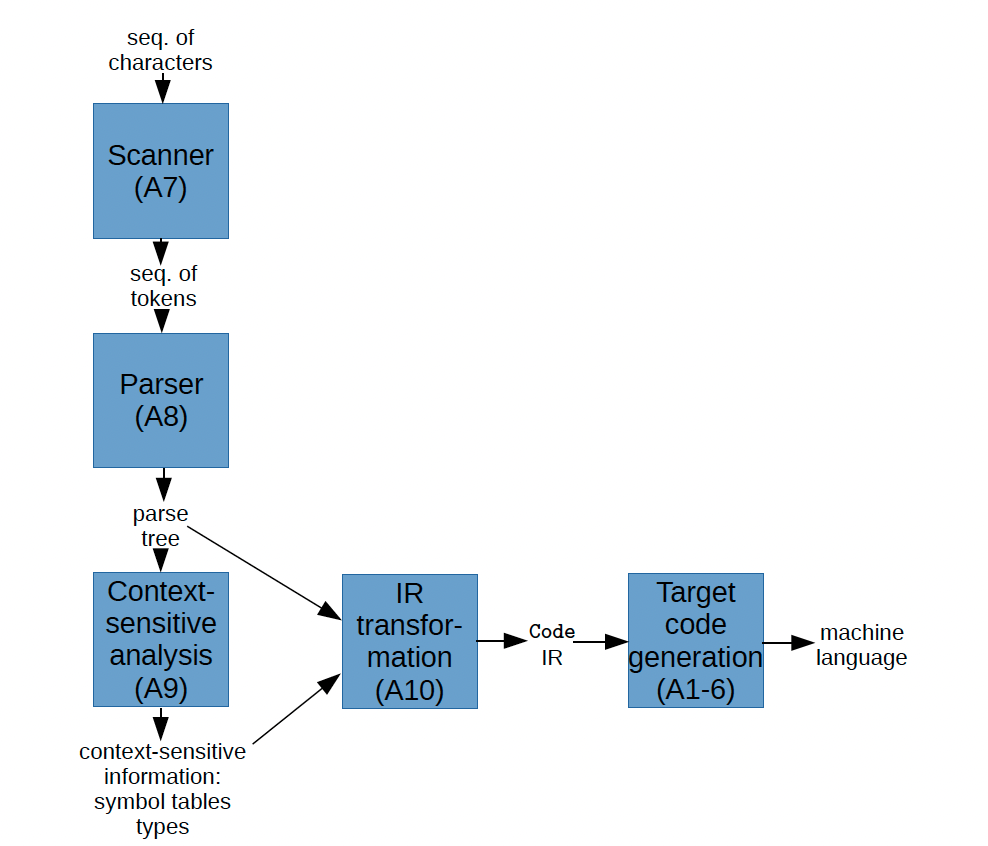# Intermediate Representation (IR) §

In a Compiler, an intermediate representation (IR) is a data structure that represents the program being compiled.

The following diagram summarizes the overall structure of a compiler (Lacs compiler):Implementation that Brian gave me because I didn’t know how to implement closures

def generateCode(procedureScope: ProcedureScope): Unit = {
val symbolTable = typedProcedures.symbolTables(procedureScope)
val opMap = Map(("PLUS", plus), ("STAR", times), ("PCT", remainder), ("MINUS", minus), ("SLASH", divide))
val cmpMap: Map[String, Label=>Code] = Map(("NE", neCmp), ("LT", ltCmp), ("GT", gtCmp), ("LE", leCmp), ("GE", geCmp), ("EQ", eqCmp))
/** Generates the Code that implements tree.
*
* This method will be called from the outside only on trees rooted at nodes of kind "expras".
* However, if it calls itself recursively on other kinds of nodes, it needs to be able to handle
* those node kinds as well.
*/
def recur(tree: Tree): Code = {
val p = tree.production
val c = tree.children
if(p == "factor ID"){
symbolTable(c(0).lhs.lexeme) match {
case Right(p) => Closure(p.procedure)
}
} else if(p == "factor NUM"){
block(LIS(Reg.result), Word(encodeUnsigned(c(0).lhs.lexeme.toInt)))
} else if(p == "factor LPAREN expr RPAREN"){
recur(c(1))
} else if(p == "factor factor LPAREN argsopt RPAREN"){
val argExprs = collect(c(2), "expr")
val args = argExprs.map(expr => recur(expr))
val params = argExprs.map(expr => new Variable("param", isPointer=typedProcedures.typeMap(expr).isInstanceOf[FunctionType]))
recur(c(0)) match {
case Closure(p) => return Call(p, args)
case _ => return CallClosure(recur(c(0)), args, params)
}
} else if (p == "term term STAR factor" || p == "term term SLASH factor" || p == "term term PCT factor" || p == "expr expr PLUS term" || p == "expr expr MINUS term") {
binOp(recur(c(0)), opMap(c(1).lhs.kind), recur(c(2)))
} else if (p == "expr IF LPAREN test RPAREN LBRACE expras RBRACE ELSE LBRACE expras RBRACE"){
ifStmt(recur(c(2).children(0)), cmpMap(c(2).children(1).lhs.kind), recur(c(2).children(2)), recur(c(5)), recur(c(9)))
} else if(p == "expras expra SEMI expras"){
block(recur(c(0)), recur(c(2)))
} else if (p == "expras expra" || p == "expra expr" || p == "expr term" || p == "term factor"){
recur(c(0))
} else if(p == "expra ID BECOMES expr") {
block(recur(c(2)), write(symbolTable(c(0).lhs.lexeme).left.get.variable, Reg.result) )
} else {
sys.error("no matching production")
}
}